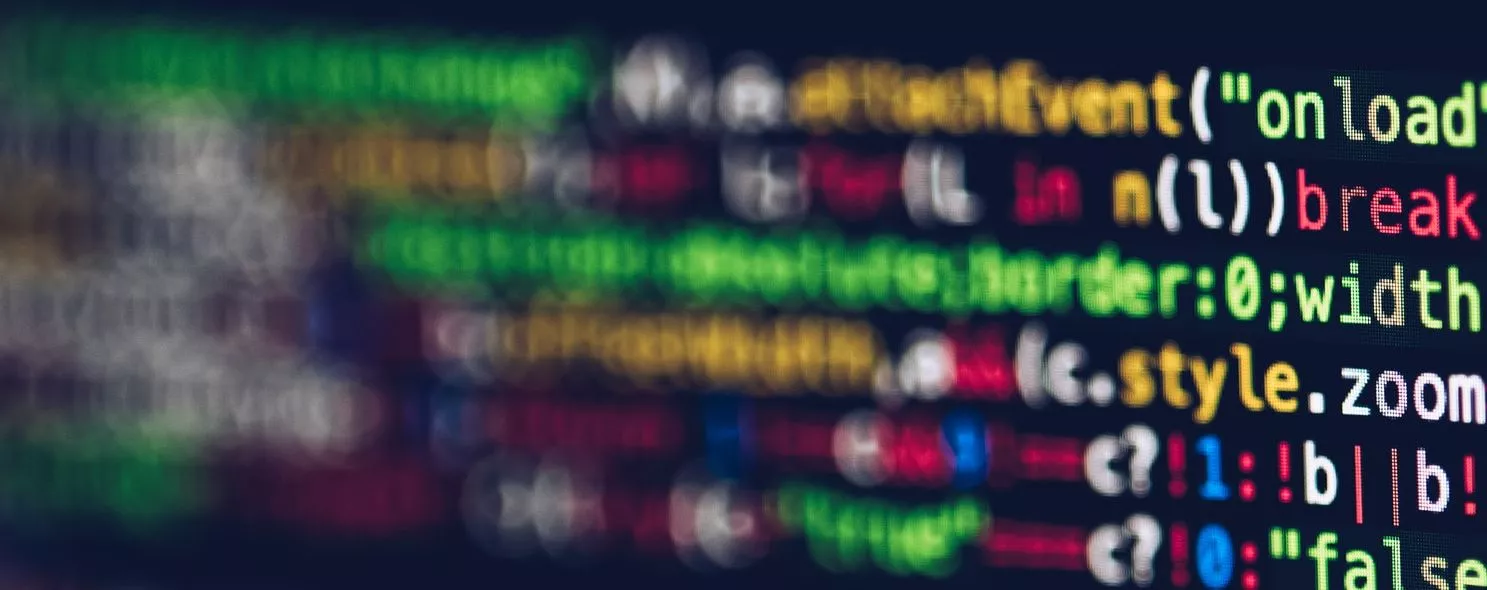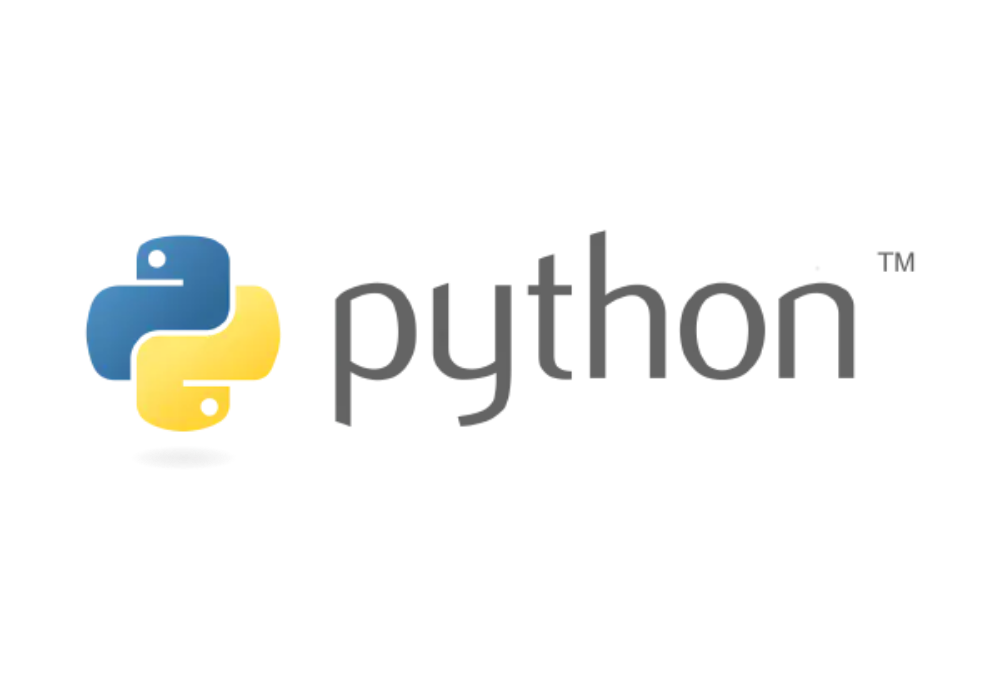Connect With Us:## Python101: 4. Flow ControlPython
by Admin/ on 02 Jul 2021

## Python101: 4. Flow Control

In the world of programming, flow control is the foundation on which programmers operate. Flow control determines the way in which programs are executed, and this section gives you an introduction to Python flow control-related syntax.

## if statement

The if statement indicates how what condition occurs and what logic is executed.

Syntax

``````if Judgment condition.
Execute the statement ......
else.
Execute the statement ......
``````

Example.

``````# x = int(input("Please enter an integer: "))
x = -5
if x < 0:
x = 0
print('Negative changed to zero')
elif x == 0:
print('Zero')
elif x == 1:
print('Single')
else:
print('More')
``````

There may be zero to multiple elif sections, and else is optional. The keyword ‘elif’ is an abbreviation for ‘else if’, which effectively avoids excessive indentation. if … elif … elif … sequence is used in place of switch or case statements in other languages.

## for loop

Python for loops can iterate over any sequence of items, such as a list or a string.

The syntax format of the for loop is as follows:

``````'''
for followed by the variable name, in followed by the sequence, note the colon
The for loop takes one value at a time from the sequence and puts it into the variable
The sequence here is mainly a list, a tuple, a string, a file
'''
for iterating_var in sequence:
statements(s)
``````

Examples are as follows:

``````for letter in 'Python': # First example
print('Current letter :', letter)

fruits = ['banana', 'apple', 'mango']
for fruit in fruits: # Second instance
print('Current letter :', fruit)

print("Good bye!")
``````

It is also possible to traverse the content by indexing the address

``````fruits = ['banana', 'apple', 'mango']
for index in range(len(fruits)):
print('Current fruit :', fruits[index])

print("Good bye!")
``````

## while loop

The while statement in Python programming is used to loop through a program, that is, to loop through a program under a certain condition in order to handle the same task that needs to be repeated. Its basic form is.

Syntax

``````while Judgment condition.
Execute the statement ......
``````

Example.

``````count = 0
while (count < 9):
print( 'The count is:', count)
count = count + 1

print("Good bye!")
``````

You can also add judgment logic to the while loop

``````count = 0
while count < 5:
print(count, " is less than 6")
count = count + 1
else:
print(count, " is not less than 6")
``````

## range() function

If you need a sequence of values, the built-in range() function comes in handy, which generates a chain of equivalence series:

Syntax

``````range (start, end, scan):
``````

Meaning of parameters.

• `start`: Count starts from start. The default is to start from 0. For example, `range(5)` is equivalent to `range(0, 5)`;
• `end`: The count ends at end, but not including end. e.g. `range(0, 5)` is `[0, 1, 2, 3, 4]` without 5
• `scan`: The spacing of each jump, default is 1. e.g. `range(0, 5)` is equivalent to `range(0, 5, 1)`

Example

``````for i in range(6):
print(i)
print(range(6),'finish')

for i in range(6,10):
print(i)
print(range(6,10),'finish')

for i in range(6,12,2):
print(i)
print(range(6,12,2),'finish')
``````

If you need to iterate over the chain index, use a combination of `range()` and `len()` as shown below:

``````a = ['i', 'love', 'coding', 'and', 'free']

for i in range(len(a)):
print(i, a[i])
``````

## break Usage

The break statement allows you to jump out of the for and while loop bodies. If you terminate from a for or while loop, any corresponding else block of the loop will not be executed.

Example

``````for letter in 'ityouknow': # First instance
if letter == 'n': # Break when letter is n
break
print ('Current letter :', letter)
``````

## continue Usage

The continue statement is used to skip the remaining statements in the current loop block and then continue to the next round of loops.

Example

``````for letter in 'ityouknow': # First instance
if letter == 'n': # Skip output when letter is n
continue
print ('Current letter :', letter)
``````

## pass statement

Python pass is an empty statement, designed to preserve the structural integrity of the program. It is used when something is syntactically necessary, but the program doesn’t do anything.

Example

``````while True:
pass # Busy-wait for keyboard interrupt (Ctrl+C)

# This is usually used to create minimally structured classes:
class MyEmptyClass:
pass
``````

## Summary

This section gives you an introduction to the flow control-related syntax of Python, which facilitates conditional control in code logic later on.

Reference